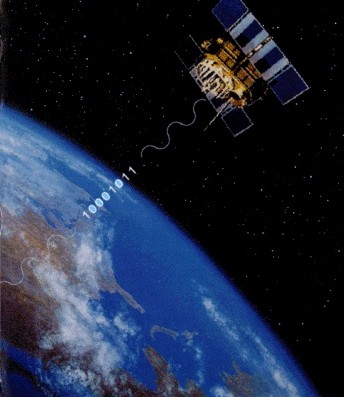How does GPS work?

• The GPS satellites send out unique and predictable signals.Figure 2.1: Satellite Signal

• The Master Control Station in Colorado Springs updates the GPS satellites with predicted positions (ephemeries) and controls the GPS time.

• The GPS observables are two carriers containing code:

• L1-Frequency (1575,42 MHz) = 19,05 cm wavelenght

• C/A-Code

• P-Code

• Carrier

• L2-Frequency (1227,60 MHz) = 24,45 cm wavelenght

• P-Code

• Carrier

Author: Michael Schulz, GEOSOFT